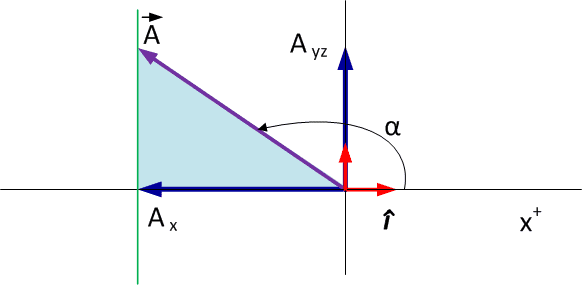# Direction of cartesian vector

## Homework Statement

if the alpha is larger than 90 degree ( which means the resultant F is lean towards -x axis , then the angle between the Ax and the line from Ax to A will be less than 90 , am i right ?

## Answers and Replies

BvU
Science Advisor
Homework Helper
No. It will be exactly 90##^\circ##

(the 90##^\circ## as indicated in figure 2-26 (a) )

No. It will be exactly 90##^\circ##

(the 90##^\circ## as indicated in figure 2-26 (a) )
why , i cant visualize it??

No. It will be exactly 90##^\circ##

(the 90##^\circ## as indicated in figure 2-26 (a) )
If the force is lean towards negative x axis , it means the angle between the force and positive x -axis is more than 90 degree, right ? then how is it possible for it to remain 90 degree?

BvU
Science Advisor
Homework Helper
You were talking about the line from Ax to A, not about the line from the origin to A (i.e. ##\vec A## itself).

You were talking about the line from Ax to A, not about the line from the origin to A (i.e. ##\vec A## itself).
ya , i mean the line from Ax to A, not about the line from the origin to A (i.e. A⃗ itself).
if the force is lean towards the negative x-axis then the , we would have three line , right , namely the line joining origin to Ax , A(which is lean towards negative x axis ) , and a line which join A to negative x-axis , right ? if it is so , then the i an undersatnd why the angle is 90 degree. if it so , the triangle formed is at opposite of the current one .

BvU
Science Advisor
Homework Helper
I think you've got it all right and correct, just that the wording in post #1 was rather unfortunate.
With nowadays visualization possibilities it's almost a stone-age approach to put things in words, but let's do it anyway:

let's look in the blue plane of figure 2-26 (a) for the case ##\alpha \in [\pi/2, \pi]## where all the angles in our story are
 I mean to say that all the angles are in that plane. Sorry for the ambiguity.​so the vertical line Ayz is in the yz plane and that plane is perpendicular to the x axis.

and as you can see the line from Ax to A (the green line) is parallel to that plane and therefore also perpendicular to the x axis. Hence my 90 ##^\circ##.

You of course in your post #1 meant to refer to the angle between ##\vec A## and ##\vec A_x## which as you can see is ##\pi-\alpha ## and thereby in the range 0 to 90##^\circ##.

Note that I forgot to draw the arrow above ##\vec A_x## and ##\vec A_{yz}##. Nobody is perfect, be we keep trying.
To be specific: in ##\vec A_x = A_x\, \hat\imath\ ##, ##\ A_x## is a number (negative for the alpha in the picture: ##A_x = |\vec A | \cos\alpha##​

Last edited:
•werson tan